### IDLE and Syntax Errors

When someone writes code in a high-level language they might make some mistakes. Look at the following Python program.

```num1 = 5
num2 = 10
c = num1 + num2 +
print(c)
```

Here, the programmer made a typographic error (a “typo”); the third statement contains an unnecessary addition operator ( + ). If you try to execute this program, Python’s interpreter displays the error “invalid syntax” as shown in Figure1.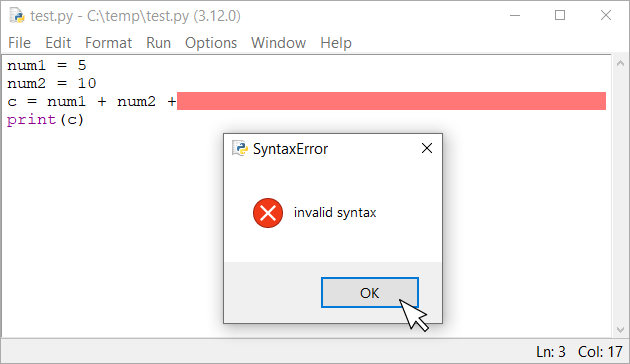Figure 1 Python displays a syntax error

Click on the “OK” button. IDLE marks the erroneous line in red. All you have to do is correct the error and try to execute the program again!

### IDLE and Runtime Errors

Now, look at the following Python program.

```num1 = float(input("Enter number A: "))
num2 = float(input("Enter number B: "))
c = num1 / num2
print(c)
```

The program may look perfect, but have you thought about the possibility of `num2` being zero? Unfortunately, the way this program is written permits the user to enter a value of zero for variable `num2`. If you try to execute this program, and enter a value of zero for `num2`, Python’s interpreter complains and displays the error “float division by zero” (Figure 2). Also, the interpreter tells you that this runtime error happened when line 3 was executed!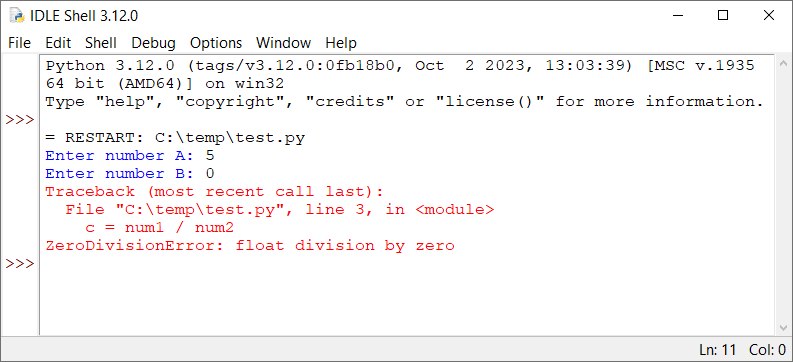Figure 2 Python throws a runtime error

Notice: Using a decision structure, the computer could determine whether it should perform this division. We won’ t deal with this problem right now! It was used just to demonstrate how IDLE displays a run-time error when it occurs.

### IDLE and Logic Errors – Debugging Step-By-Step

Compared to syntax errors, logic errors are more difficult to find. Since IDLE cannot spot and mark logic errors, you are all alone! Let’s look at the following Python program, for example. It prompts the user to enter two numbers and calculates and displays their sum. However, it contains a logic error!

```a = int(input("Enter 1st value: "))
b = int(input("Enter 2nd value: "))
Sl = a + b
S1 = Sl ** 2
print("The square of sum is:", Sl)
```

If you type this program into IDLE and execute it, you will notice that not a single line is marked in red, indicating any error. However, if you run the program and enter two values, 2 and 3, you will see for yourself that even though the square of the sum of 2 and 3 is 25, IDLE insists that it is 5, as shown in Figure 3.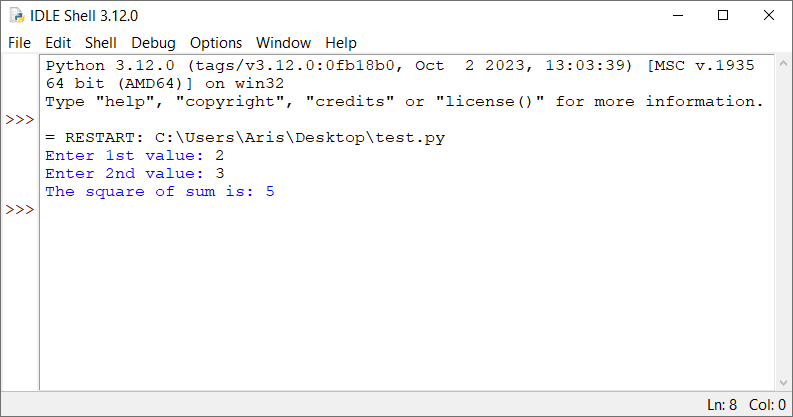Figure 3 Viewing the result of a logic error in IDLE

What the heck is going on? Of course, for an expert programmer, correcting this error would be a piece of cake. But for you, a novice one, even though you are trying hard you find nothing wrong. So, where is the error?

Sometimes human eyes get so tired that they cannot see the obvious. So, let’s try to use some magic! Let’s try to execute the program step by step using the debugger. This gives you the opportunity to observe the flow of execution and take a closer look at the current values of variables in each step.

To open the debugger, you need to select “Debug→Debugger” from the main menu of the IDLE Shell. The popup window that appears should look like the one shown in Figure 4.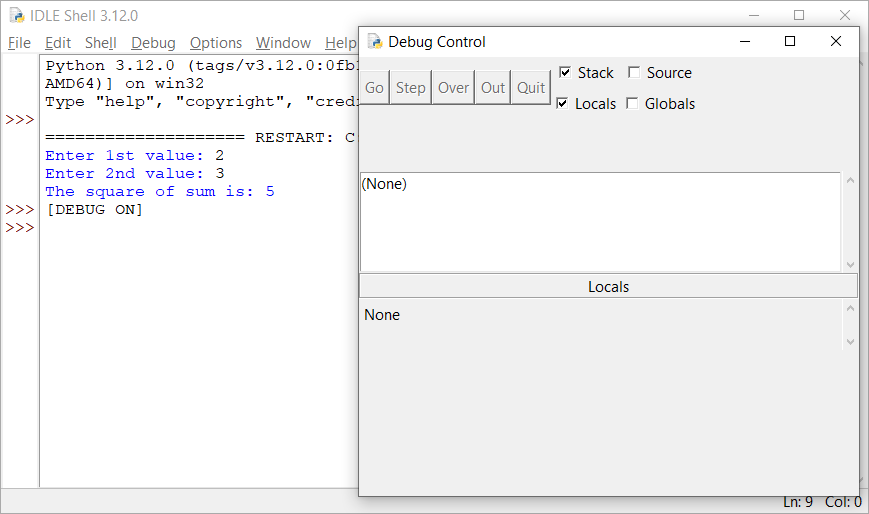Figure 4 The debugger’s window

Execute the program again. The debugger displays the message shown in Figure 5.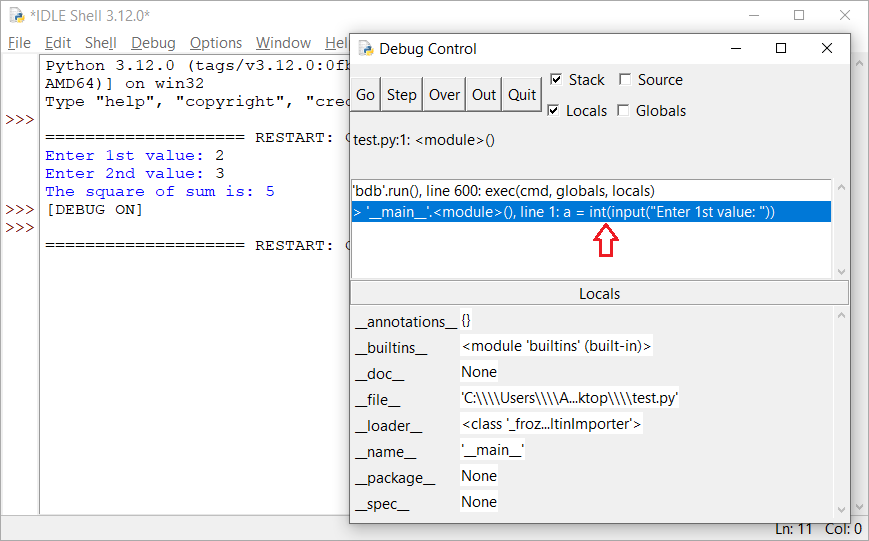Figure 5 The debugger’s window right after starting the execution of a program

In this step, the first statement of the program is not yet executed. Click on the “Over” button in the debugger’s window to execute the first statement. The debugger’s window, after executing the first statement, is shown in Figure 6.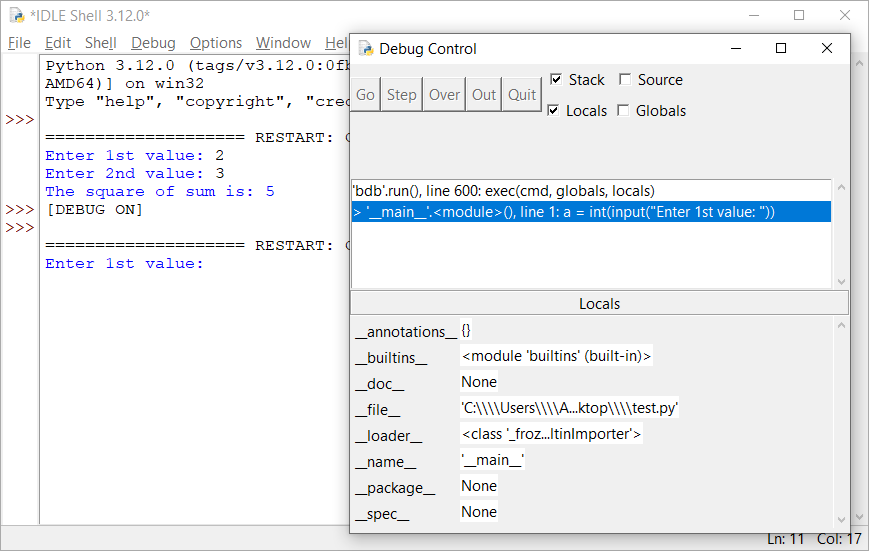Figure 6 The debugger’s window right after executing the first statement

In this step, the program waits for you to enter a value. Place the cursor inside the IDLE Shell, type the value 2, and hit the “Enter ⤶” key.

Now, look at the debugger’s window (Figure 7). It displays the next statement to be executed, which is the statement `b = int(input(Enter 2nd value: ))` and below (in Locals), it displays the current value of variable `a`.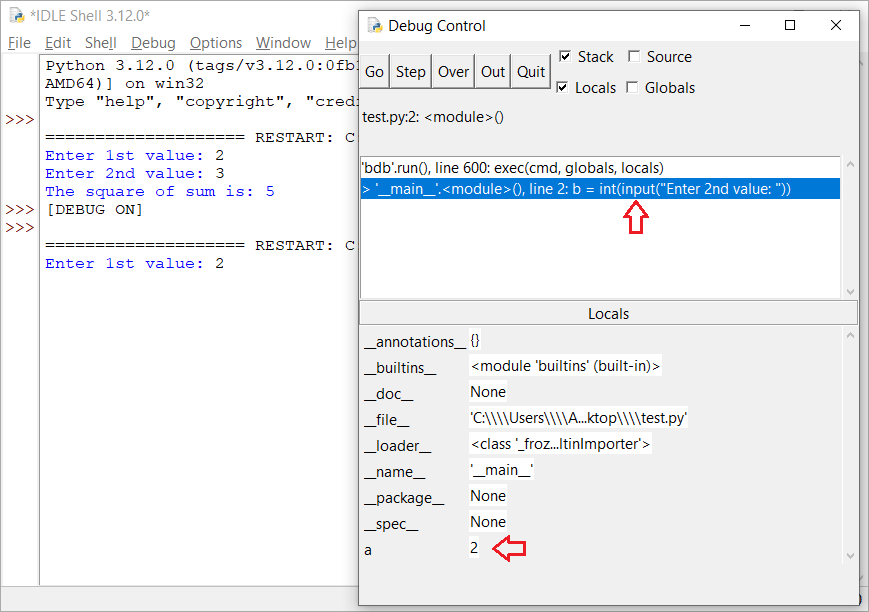Figure 7 The debugger’s window after entering the first value

Click on the “Over” button again. This action executes the second statement. Place the cursor inside the IDLE Shell, type the value 3, and hit the “Enter ⤶” key.

Click on the “Over” button again. The third statement `Sl = a + b` is executed.

Click on the “Over” button once again to execute the fourth statement `S1 = Sl ** 2`. What you expected here was the sum of 2 and 3, which is 5, to be squared and assigned back to variable `Sl`. Instead of this, the value 25 is assigned to the variable `S1`, as shown in Figure 8.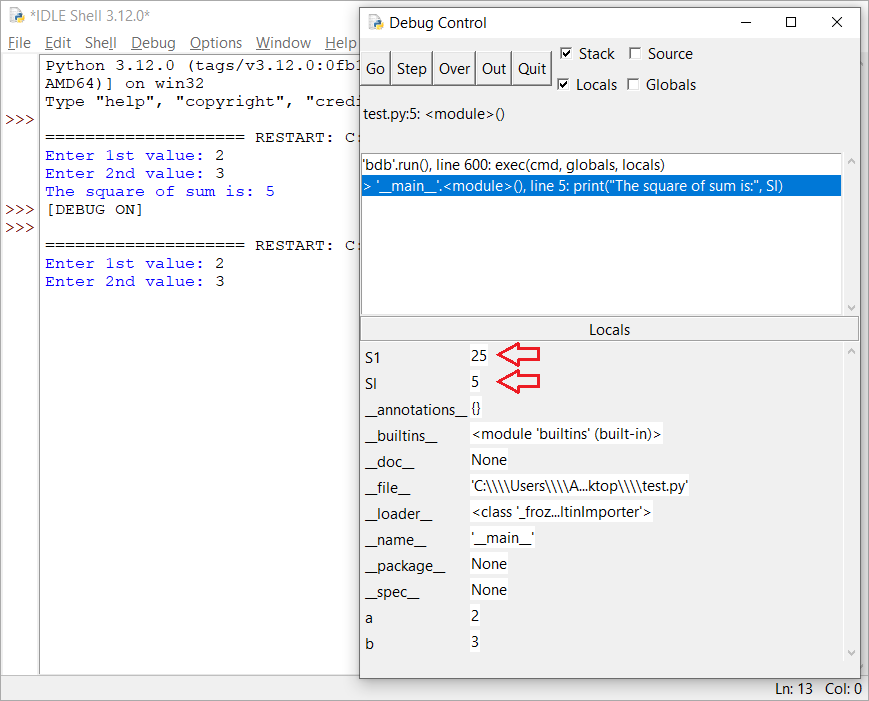Figure 8 All variables are displayed in the “Locals” section of the debugger’s window.

Notice: Variable `Sl` is different from `S1`. The first one uses the characters S and L (in lowercase), while the second one uses the characters S and 1 (digit one).

Now it becomes more obvious to you! You mistakenly declared two variables in the main memory (RAM), the variable `Sl` with a lowercase L and the variable `S1` with a a digit 1. So, when the flow of execution goes to the last statement, `print("The square of sum is:", Sl)` the value 5 instead of the value 25 is displayed.

Congratulations! You have just found the error! Click on the “Quit” button in the debugger’s window to cancel execution, correct the error by changing variable S1 to Sl, and you are ready! You just performed your first debugging! Re-execute the program and you will now see that it calculates and displays the square of the sum correctly.

### Debugging logic errors by adding breakpoints

Debugging step by step has a big disadvantage. You have to click on the “Over” button again and again until you reach the position where the error might be. Can you imagine yourself doing this in a large program?

For large programs there is another approach. If you suspect that the error is somewhere at the end of the program there is no need to debug all of it right from the beginning. You can add a marker (called a “breakpoint”) near where you think that the error might be, execute the program and when flow of execution reaches that breakpoint, the flow of execution will pause automatically. You can then take a closer look at the current values of the variables at the position where the program was paused.

Notice: When the program pauses, you have two options for resuming the execution: you can add a second breakpoint somewhere below in the program, click on the “Go” button, and allow the program to continue execution until that new breakpoint; or, you can just use the “Over” button and execute the program step by step thereafter.

The next Python program prompts the user to enter two values and then it calculates their sum, their difference, and their average value.

However, the program contains a logic error. When the user enters the values 10 and 12, the value 16, instead of 11, is displayed as the average.

```a = float(input("Enter 1st value: "))
b = float(input("Enter 2nd value: "))
s = a + b
d = a - b
average = a + b / 2
print("Sum:", s)
print("Difference:", d)
print("Average:", average)
```

You suspect that the problem is somewhere near the end of the program. However, you do not want to debug the entire program, but just the portion in which the error might be. So, let’s try to add a breakpoint at the `d = a - b` statement (see Figure 9). Right click on that statement and select “Set Breakpoint”.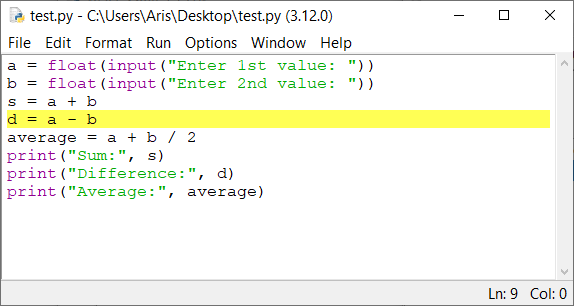Figure 9 Adding a breakpoint to a program

Notice: You know that a breakpoint has been set when the corresponding code line is marked in yellow.

Make sure that the “Debugger” is open. If not, open it by selecting “Debug→Debugger” from the main menu of the IDLE Shell.

Run the program and then, click on the “Go” button in the debugger’s window. Enter the values 10 and 12 when requested in the IDLE Shell. You will notice that just after you enter the second number and hit the “Enter ⤶” key, the flow of execution pauses at the breakpoint.

Now you can take a closer look at the current values of the variables. Variables `a`, `b`, and `s` contain the values 10.0, 12.0, and 22 respectively, as they should, so there is nothing wrong so far, as shown in Figure 10.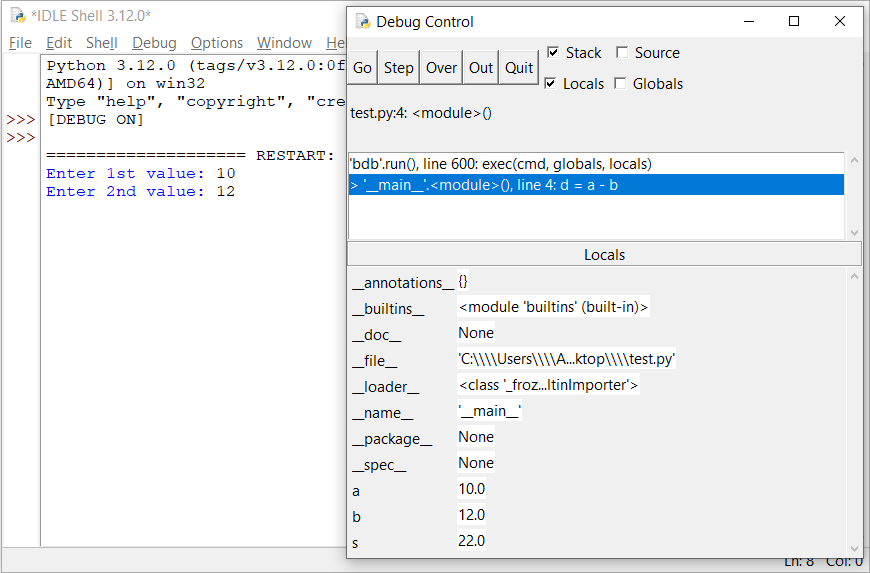Figure 10 Viewing the current values of the variables in the debugger’s window

Click on the “Over” button once. The statement  `d = a − b` executes and variables `a`, `b`, `d` and `s` contain the values 10.0, 12.0, −2.0, and 22.0 respectively, as they should, so there is still nothing wrong so far, as shown in Figure 11.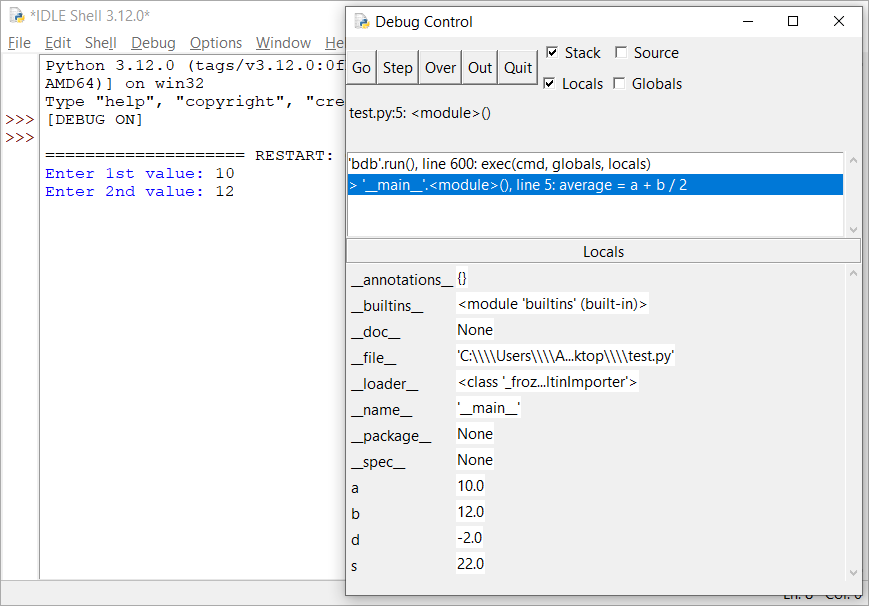Figure 11 Viewing the current values of the variables in the debugger’s window

Click on the “Over” button once again. The statement `average = a + b / 2` executes and variables `a`, `average`, `b`, `d`, and `s` contain the values 10.0, 16.0, 12, −2, and 22 respectively, as shown in Figure 12.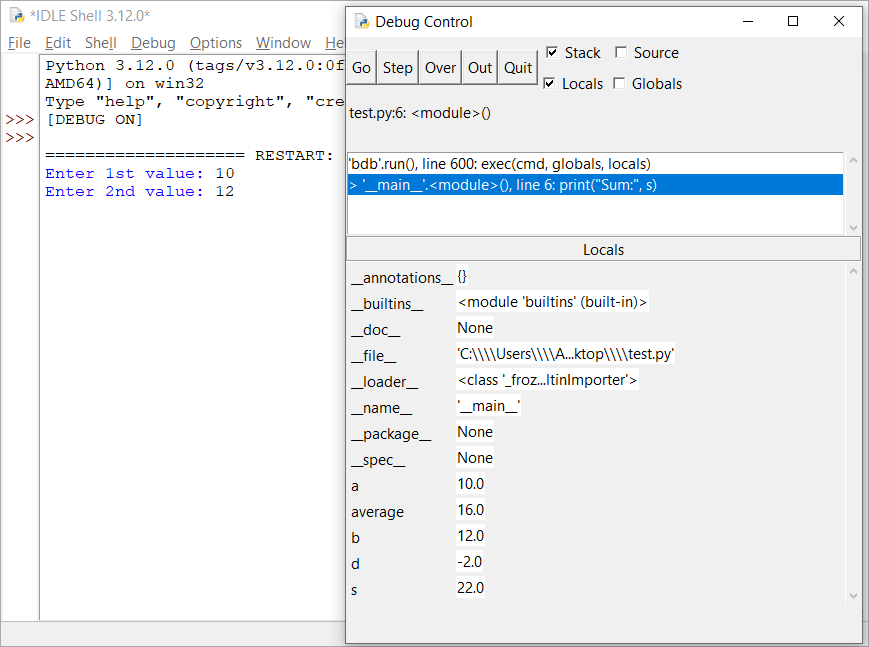Figure 12 Viewing the current values of the variables in the debugger’s window

There it is! You just found the statement that erroneously assigns a value of 16.0, instead of 11.0, to the variable average! And now comes the difficult part; you should consider why this happens!

After two full days of thinking, it becomes obvious! You had just forgotten to enclose `a + b` inside parentheses; thus, only the variable `b` was divided by 2. Click on the “Quit” button in the debugger’s window, remove all breakpoints, correct the error by enclosing `a + b` inside parentheses and you are ready! Re-execute the program and see now that it calculates and displays the average value correctly.

Notice: You can remove a breakpoint the same way you added it: right click on the corresponding code line, and select “Clear Breakpoint”.